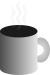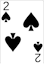#Coffee SpaceListen:

## Micro-Code

### Background

These programs have been designed to be written on the back of a business card, with their output displayed on the front.

The following is the limitations on the design:

• Compile using strict C90
• Include comments for operation where possible
• Limitation on 80x25 characters

There are the following assumptions that are reasonable to make:

• Running a modern Linux OS
• Compiling with GCC

### Pattern Test

#### Numbers

• With comments: 254 characters (not including newlines)
• Without comments: 201 characters (not including newlines)

#### Code

```0001 #include<stdio.h>/*gcc a.c; ./a.out; eog b.ppm*/
0002 main(){int w=256,h=256,k;FILE*f=fopen("b.ppm","w");fprintf(f,"P6\n%d %d\n255\n"
0003 ,w,h);for(;--h>=0;){for(k=w;k>=0;--k){char c={h%256,(k*h)%256,k%256};fwrite(
0004 c,1,3,f);}}fclose(f);}/*coffeespace.org.uk*/```

#### Use

To compile:

`0005 gcc a.c`

To run:

`0006 ./a.out`

To view:

`0007 eog b.ppm`

This program scales well by changing what `w` and `h` equal, which are width and height respectively. Numbers that reflect `2^X` display nicer than others.

### Mandelbrot

#### Numbers

• Without comments: 291 characters (not including newlines)

#### Code

```0008 #include<stdio.h>
0009 main(){int k=256,w,h=k,x,a=k/2;double b=a/2,i,j,y,z,t;FILE*f=fopen("b.ppm","w")
0010 ;fprintf(f,"P6\n%d %d\n255\n",k,k);for(;h--;)for(w=k;w--;){i=j=0;y=(w-a)/b;z=(h
0011 -a)/b;x=k;for(;i*i+j*j<4&&x--;){t=i*i-j*j+y;j=2*i*j+z;i=t;}char c={x<<4,x<<2
0012 ,x};fwrite(c,1,3,f);}fclose(f);}```

#### Use

To compile:

`0013 gcc a.c`

To run:

`0014 ./a.out`

To view:

`0015 eog b.ppm`

The image produced is really good looking and well detailed with `k = 256^X` where `X` is greater than 1. It has been tested with `k = 2^14 = 16385`, on a 3rd generation i5 processor, taking about 3 or 4 hours. This program certainly comes under the category of “embarrassingly parallel” and could benefit from multi-core processing, as little RAM and disk speed is used and pixels can be calculated independently.

### Difference

#### Output

FILE_1 (`t1.txt`)

```0016 Hello World!
0017 1234567890
0018 Difference
0019 0123456789
0020 hello```

FILE_2 (`t2.txt`)

```0021 Hello World!
0022 1234567890
0023 difference
0024 9876543210
0025 hello
0026 extra```

Output

```0027 
0028 t1.txt
0029 Difference
0030
0031 t2.txt
0032 difference
0033
0034 
0035 t1.txt
0036 0123456789
0037
0038 t2.txt
0039 9876543210
0040
0041 
0042 t2.txt
0043 extra```

#### Numbers

• Without comments: 383 characters (not including newlines)

#### Code

```0044 #include<stdio.h>
0045 main(int c,char**v){int n=512,i=1,j,d=i,e=i;char a[n];char b[n];FILE*y=fopen(v[
0046 1],"r");FILE*z=fopen(v,"r");for(;d&&e;++i){d=fgets(a,n,y);e=fgets(b,n,z);for
0047 (j=-1;++j<n&&a[j]==b[j];)if(a[j]==0)j=n;if(j<n){char c={'[',(i/1000)+48,(i/
0048 100%10)+48,(i/10%10)+48,(i%10)+48,']',0};puts(c);if(d){puts(v);puts(a);}if(e
0049 ){puts(v);puts(b);}}}fclose(y);fclose(z);}```

#### Use

To compile:

`0050 gcc a.c`

To run:

`0051 ./a.out FILE_1 FILE_2`

To view, read the terminal output.